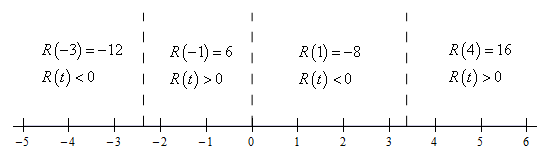Paul's Online Notes
Home / Calculus I / Review / Functions
Show Mobile Notice Show All Notes Hide All Notes
Mobile Notice
You appear to be on a device with a "narrow" screen width (i.e. you are probably on a mobile phone). Due to the nature of the mathematics on this site it is best views in landscape mode. If your device is not in landscape mode many of the equations will run off the side of your device (should be able to scroll to see them) and some of the menu items will be cut off due to the narrow screen width.

### Section 1.1 : Review : Functions

28. Find the domain of $$\displaystyle P\left( t \right) = \frac{{5t + 1}}{{\sqrt {{t^3} - {t^2} - 8t} }}$$.

Show All Steps Hide All Steps

Hint : We need to avoid negative numbers under the square root and because the quantity under the root is a polynomial we know that it can only change sign if it goes through zero and so we first need to determine where it is zero.
Start Solution

In this case we need to avoid square roots of negative numbers and because the square root is in the denominator we’ll also need to avoid division by zero issues. We can satisfy both needs by requiring,

${t^3} - {t^2} - 8t = t\left( {{t^2} - t - 8} \right) > 0$

Note that there is nothing wrong with the square root of zero, but we know that the square root of zero is zero and so if we require that the polynomial under the root is strictly positive we’ll know that we won’t have square roots of negative numbers and we’ll avoid division by zero.

Now, despite the fact that we need to avoid where the polynomial is zero we know that it will only change signs if it goes through zero and so we’ll next need to determine where the polynomial is zero.

Clearly one value is $$t = 0$$ and because the quadratic does not factor we can use the quadratic formula on it to get the following two additional points.

\begin{align*}t & = \frac{{1 \pm \sqrt {{{\left( { - 1} \right)}^2} - 4\left( 1 \right)\left( { - 8} \right)} }}{2} = \frac{{1 \pm \sqrt {33} }}{2} & \hspace{0.25in}\hspace{0.25in} & t = \frac{{1 + \sqrt {33} }}{2} = 3.372281\\ & & \hspace{0.25in}\hspace{0.25in} & t = \frac{{1 - \sqrt {33} }}{2} = - 2.372281\end{align*}

So, these three points ($$t = 0$$, $$t = - 2.372281$$ and $$t = 3.372281$$ are the only places that the polynomial under the root can change sign.

Hint : Because the polynomial can only change sign at these points we know that it will be the same sign in each region defined by these points and so all we need to know is the value of the polynomial as a single point in each region.
Show Step 2

Here is a number line giving the value/sign of the polynomial at a test point in each of the region defined by these three points. To make it a little easier to read the number line let’s define the polynomial under the radical to be,

$R\left( t \right) = {t^3} - {t^2} - 8t = t\left( {{t^2} - t - 8} \right) > 0$

Now, here is the number line,Hint : Now all we need to do is write down the values of $$x$$ where the polynomial under the root will be positive (recall we need to avoid division by zero) and we’ll have the domain.
Show Step 3

The domain will then be all the points where the polynomial under the root is positive, but not zero as we also need to avoid division by zero, and so the domain is,

${\rm{Domain : }}\frac{{1 - \sqrt {33} }}{2} < t < 0,\,\,\,\frac{{1 + \sqrt {33} }}{2} < t < \infty$